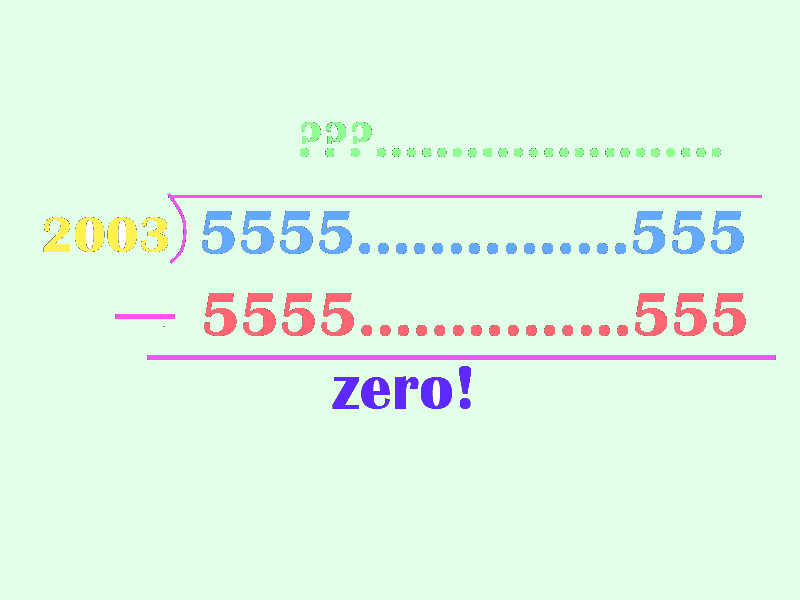# Too many 5's

Algebra Level 5There is a positive integer $x$, such that all digits of $x$ are $5$.

The remainder obtained on dividing $x$ by $2003$ is $0$.

Find the last three digits of the quotient thus obtained.

×

Problem Loading...

Note Loading...

Set Loading...